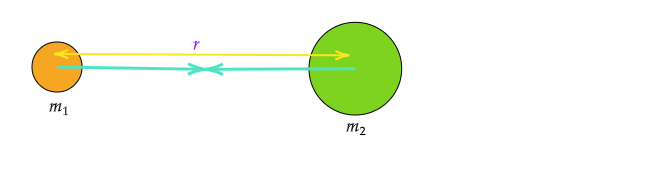# Derive the formula for the universal law of gravitation.

Newton's law of universal gravitation:
Newton's law of gravitation, states that every particle of matter in the universe attracts every other particle in the universe with a force varying directly as the product of their masses and inversely as the square of the distance between the particles.Let there are two objects of mass $m_1$ and $m_2$ and the distance between the objects is $r$, then according to law of universal attraction, the attraction between the objects

$F\propto m_1.m_2$

And $F\propto \frac{1}{r^2}$

Therefore, $F\propto\frac{m_1.m_2}{r^2}$

Or $F=G\frac{m_1.m_2}{r^2}$, Where $G$ is constant whose value is $6.67\times10^{-11}$.

Let $m$ be the mass of an object and $M$ be the mass of the earth and $g$ be the gravity.

Then weight$=mg$

$mg=G\frac{mM}{r^2} Or$g=G\frac{M}{r^2}\$.

Updated on: 10-Oct-2022

764 Views